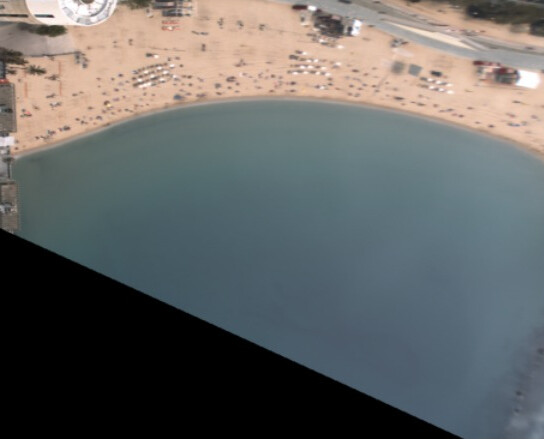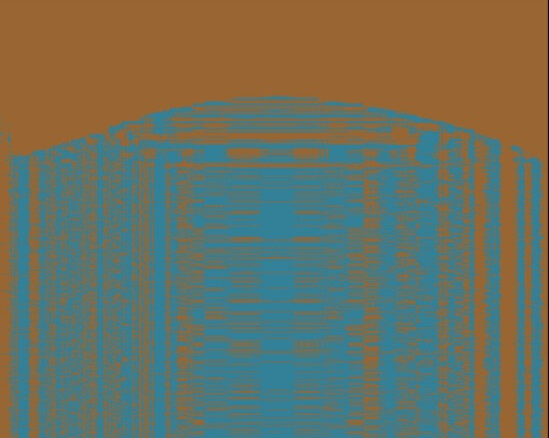# Improve LSTM classification network

Hi, I am training a network to detect the shorelines in aereal images. The idea of my project is not use the typical convolutional network, and instead of this, I am using an LSTM to process column of the images and then classify them between land (0) and sea (1), to train something similar to a segmentation network.

Right now my network is like this:

``````class BiLSTM(nn.Module):
def __init__(self, input_size, hidden_size, num_classes, num_layers):
super(BiLSTM, self).__init__()
self.hidden_size = hidden_size

self.lstm = nn.LSTM(input_size=input_size, hidden_size=hidden_size, num_layers=num_layers, bidirectional=True)
self.hidden2line = nn.Linear(603, 603)
self.relu = nn.ReLU()
self.upsample = nn.Upsample(size=603)

def forward(self, input_col):
lstm_out, (hn, cn) = self.lstm(input_col.float())
out = self.upsample(lstm_out)
out = self.hidden2line(out[:, -1, :])
out = self.relu(out)

return out

def backward(self, loss):
loss.backward()
``````

I feed columns of the image to the LSTM network, then I upsample them to be equal size to the column size, then I use the linear layer, and then a ReLu.

The output of the network is a vector of equal size of the column length, containing 0 or 1, depending of if it is land or sea.

After a few tests, I am getting “pretty good” results after 100 epochs, with a learning rate of 0.001, getting a MSE loss of 0.005 on test dataset.

But I am very newbie to deep learning, and I am seeking ways to improve the network, how can I try to improve the network?

This is an image passed to the network, and the result:Up, has anyone any tips so I can improve my neural network?

I insist I am very newbie to this, and I would like to learn as much as I can, thank you!